### Practice Set 3.3 Polynomials Class 9th Mathematics Part I MHB Solution

Polynomials

Class 9th Mathematics Part I MHB Solution

###### Practice Set 3.3

Question 1.

Divide each of the following polynomials by synthetic division method and also by linear division method. Write the quotient and the remainder.

(2m2 – 3m + 10) ÷ (m – 5)

The linear division method is as follows: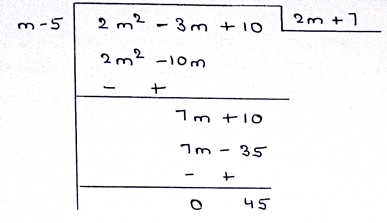Representing the polynomial in first polynomial in coefficient form:

⟹ 2.m2 – 3.m + 10

⟹ (2, – 3, 10)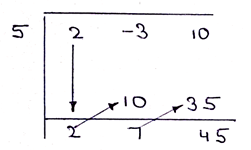Bring the first term as it is. Then multiply 5 with the value written in the bottom result area. Continue this until done.

Quotient = 2m + 7

Remainder = 45

Question 2.

Divide each of the following polynomials by synthetic division method and also by linear division method. Write the quotient and the remainder.

(x4 + 2x3 + 3x2 + 4x +5) ÷ (x + 2)

The linear division is as follows: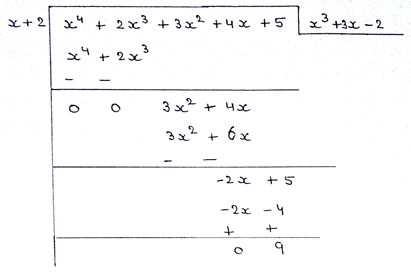Representing the polynomial in first polynomial in coefficient form:

⟹ 1.x4 + 2.x3 + 3x2 + 4x + 5

⟹ (1 2 3 4 5)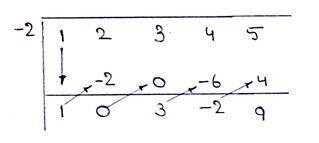So the final answer is written in the following form:

Quotient = x3 + 3x – 2

Remainder = 9

Question 3.

Divide each of the following polynomials by synthetic division method and also by linear division method. Write the quotient and the remainder.

(y3 – 216) ÷ (y – 6)

The linear division method is as follows: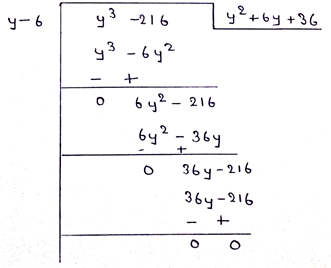Representing the polynomial in first polynomial in coefficient form:

⟹ 1.y3 + 0.y2 + 0.y – 216

⟹ (1, 0, 0, -216)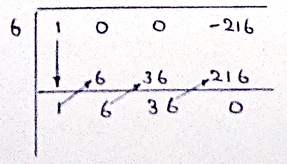So the final answer is written in the following form:

Quotient = y2 + 6y + 36

Remainder = 0

Question 4.

Divide each of the following polynomials by synthetic division method and also by linear division method. Write the quotient and the remainder.

(2x4 + 3x3 + 4x - 2x2) ÷ (x+3)

The linear division is as follows: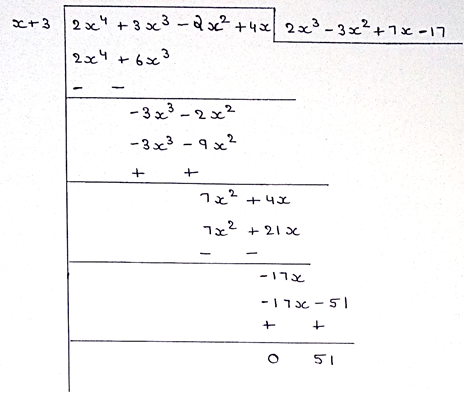Representing the polynomial in first polynomial in coefficient form:

⟹ 2.x4 + 3.x3 – 2.x2 + 4.x

⟹ (2, 3, -2, 4, 0)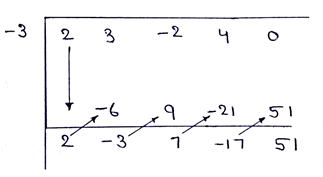So, the final answer is written in the following form:

Quotient = 2x3 – 3x2 + 7x – 17

Remainder = 51

Question 5.

Divide each of the following polynomials by synthetic division method and also by linear division method. Write the quotient and the remainder.

(x4 – 3x2 – 8) ÷ (x+4)